# Length Contraction Ether wind

unscientific
Length Contraction "Ether wind"

## Homework Statement

Due to the ether wind, anything that moves along it is "contracted".

## The Attempt at a Solution

Taking L1 to be contracted length,

(L1)2
= (L1x)2 + (L1Y)2
= (L10,X/γ)2 + (L10,Y)2

But what they wrote is the opposite..

#### Attachments

•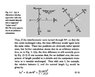contraction1.jpg
52.8 KB · Views: 359
Last edited:

Homework Helper
Gold Member

The equation (3-3) in the book is an expression for the "normal" (not contracted) length in terms of the components of the contracted length. Your expression is for the contracted length in terms of the components of the normal length.

unscientific

The equation (3-3) in the book is an expression for the "normal" (not contracted) length in terms of the components of the contracted length. Your expression is for the contracted length in terms of the components of the normal length.

Are both equivalent??

I tried to prove but the square roots got me..

unscientific
Michelson Morley Experiment: Rotating setup

## Homework Statement

Apparently rotating the setup at an angle doesn't change the time difference between t1 and t2..

## The Attempt at a Solution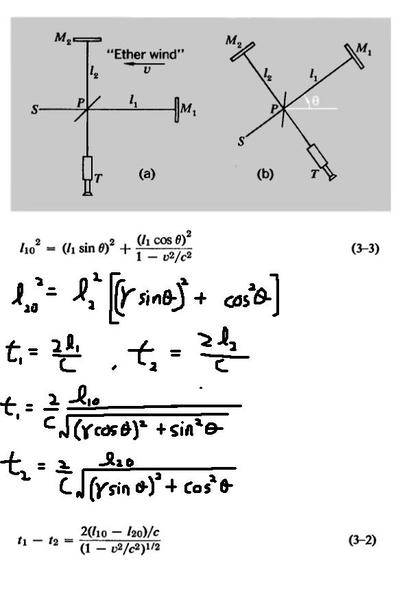But the square roots below are clearly different..

Homework Helper

square roots below what?

unscientific

square roots below what?

I tried changing everything in the square roots to only 'sin' or 'cos' but can't seem to remove the square roots at all..

Homework Helper

I'll have to see your working - is there an attachment missing?
OH I see ... there is a tinypic.com link that is not rendering for me.

unscientific

bumpp

Homework Helper

Oh there you are - couldn't access the thread for a while there.
I'll have a proper look when I have a bit of time.
Is this the reworking of the MM experiment using SR?

Last edited:
Homework Helper
Gold Member

unscientific,

Note that the time, $t_1$, to travel out and back along $l_1$ is not $2l_1/c$ because ##c## is not the speed of light relative to the apparatus. You're going to have to figure out the speed of the light relative to the apparatus for the light going out along arm $l_1$ and the (different) speed for the light coming back. Likewise for the other arm.

Last edited:
unscientific

unscientific,

Note that the time, $t_1$, to travel out and back along $l_1$ is not $2l_1/c$ because ##c## is not the speed of light relative to the apparatus. You're going to have to figure out the speed of the light relative to the apparatus for the light going out along arm $l_1$ and the (different) speed for the light coming back. Likewise for the other arm.

I assume:
1) only the component parallel to ether wind is affected by it
2) The light is aimed at a smaller angle to compensate for this

so the speed towards is:
√[ (c sinθ)2 + (c cosθ - v)2 ]

and the speed back is:

√[ (c sinθ)2 + (c cosθ + v)2 ]

Not sure if that's right..

Homework Helper
Gold Member

so the speed towards is:
√[ (c sinθ)2 + (c cosθ - v)2 ]

That would be ok if θ here is the angle that the light is "aimed at". But in that case, it's not the angle of inclination of the arm as shown in the diagram.

unscientific

That would be ok if θ here is the angle that the light is "aimed at". But in that case, it's not the angle of inclination of the arm as shown in the diagram.

I'm not sure what you mean..If c is indeed affected by ether wind won't θ be off-balanced?

Homework Helper
Gold Member

I'm not sure what you mean..If c is indeed affected by ether wind won't θ be off-balanced?
Indeed. You'll have to fire the light beam in some direction $\phi$ so that the wind will blow it into the direction θ (along the arm). I was interpreting the direction "aimed at" as the direction fired ($\phi$). Your expression for the light speed relative to the apparatus is ok if your θ is replaced by $\phi$.

You might try drawing a velocity addition triangle and using the law of cosines to express the light speed relative to the apparatus in terms of θ without worrying about the value of $\phi$.

harrylin

I assume:
1) only the component parallel to ether wind is affected by it[..]
If I correctly understand what you say, then you are copying an error of Michelson! See:

1. http://en.wikisource.org/wiki/The_Relative_Motion_of_the_Earth_and_the_Luminiferous_Ether :
" If, however, the light had traveled in a direction at right angles to the Earth's motion it would be entirely unaffected."

I think that this is also what you are saying now.

2. http://en.wikisource.org/wiki/Influence_of_Motion_of_the_Medium_on_the_Velocity_of_Light :

"In deducing the formula for the quantity to be measured, the effect of the motion of the Earth through the ether on the path of the ray at right angles to this motion was overlooked." [etc]

Does that help?

unscientific

Indeed. You'll have to fire the light beam in some direction $\phi$ so that the wind will blow it into the direction θ (along the arm). I was interpreting the direction "aimed at" as the direction fired ($\phi$). Your expression for the light speed relative to the apparatus is ok if your θ is replaced by $\phi$.

You might try drawing a velocity addition triangle and using the law of cosines to express the light speed relative to the apparatus in terms of θ without worrying about the value of $\phi$.

Can't seem to get it to be independent of ∅... it turns out as (θ-∅)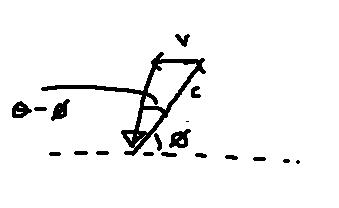unscientific

Indeed. You'll have to fire the light beam in some direction $\phi$ so that the wind will blow it into the direction θ (along the arm). I was interpreting the direction "aimed at" as the direction fired ($\phi$). Your expression for the light speed relative to the apparatus is ok if your θ is replaced by $\phi$.

You might try drawing a velocity addition triangle and using the law of cosines to express the light speed relative to the apparatus in terms of θ without worrying about the value of $\phi$.

I think this question works on the assumption that speed of light 'c' is unaffected by aether. Rather, they are trying to do length contraction here...

This brings me to my second post:

## Homework Statement

Apparently rotating the setup at an angle doesn't change the time difference between t1 and t2..

## The Attempt at a SolutionBut the square roots below are clearly different..

Homework Helper
Gold Member

But all I'm saying is that if you let c' represent the speed of light along the arm ##l_1##, then you can express c' in terms of c and θ using relative velocity arguments. See the attached diagram.

#### Attachments

Last edited:
unscientific

I haven't yet had time to look carefully at your links. They look interesting! But all I'm saying is that if you let c' represent the speed of light along the arm ##l_1##, then you can express c' in terms of c and θ using relative velocity arguments. See the attached diagram.

In this part they assume speed of light 'c' is the same. They are assuming only the distance contracts..

This is from A.P. French's Relativity text...chapter 3. I'm finding this text very very difficult, especially chapter 2 and 3 (I've skipped the entire chapter 2) I've already completed the homework problems from Halliday, Resnick and Walker and I find them to be much easier. This is demoralizing..

Homework Helper
Gold Member

French's exercise is a little tedius to carry through, but it will work out.

What you want to show is that the difference in round trip time for the two arms will be the same no matter how you rotate the apparatus if you postulate length contraction in the ether wind. So, you still have the effect of the ether wind. Relative to the apparatus, the speed of light, c', will not be equal to the speed relative to the ether, c. Use the velocity triangle and the law of cosines as shown in the attachment to express c' in terms of c, v, and θ.

#### Attachments

Homework Helper
Gold Member

In this part they assume speed of light 'c' is the same. They are assuming only the distance contracts..
No, French is still taking into account that the speed of light relative to the apparatus will be affected by the ether wind. Note how he uses equations (3-1) which are the same as (2-9) and (2-10). But he is now adding an additional effect: the Lorentz-FitzGerald contraction. With the contraction effect added, there will be no fringe shift as the apparatus is rotated relative to the direction of the ether wind.
I've already completed the homework problems from Halliday, Resnick and Walker and I find them to be much easier. This is demoralizing..
French's exercise is a step up from Halliday. It's a rather challenging exercise, so expect a little pain.You should feel good about completing the Halliday exercises and I'm sure you'll make it through French's exercise if you stick with it.

unscientific

No, French is still taking into account that the speed of light relative to the apparatus will be affected by the ether wind. Note how he uses equations (3-1) which are the same as (2-9) and (2-10). But he is now adding an additional effect: the Lorentz-FitzGerald contraction. With the contraction effect added, there will be no fringe shift as the apparatus is rotated relative to the direction of the ether wind.

French's exercise is a step up from Halliday. It's a rather challenging exercise, so expect a little pain.You should feel good about completing the Halliday exercises and I'm sure you'll make it through French's exercise if you stick with it.

Ok, thank you for guiding me! I will give it another go!

unscientific

I worked it out and got some crazy-*** square roots...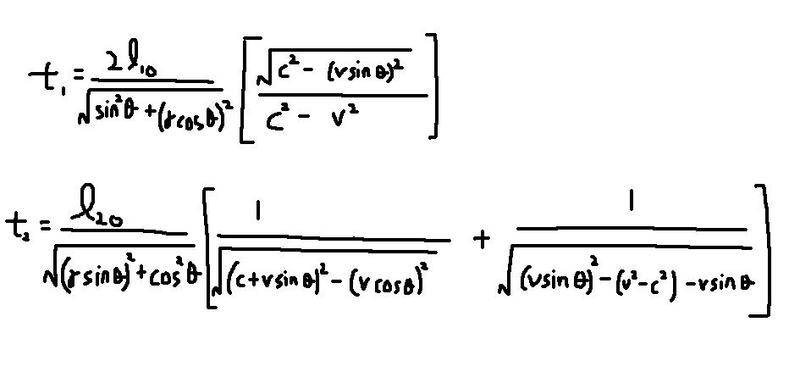Homework Helper
Gold Member

Good. Looks like your expression for t1 is correct. It will simplify.

For t2, I believe your expression in the square bracket needs some corrections.

unscientific

Good. Looks like your expression for t1 is correct. It will simplify.

For t2, I believe your expression in the square bracket needs some corrections.

First pic shows the trip towards the mirror: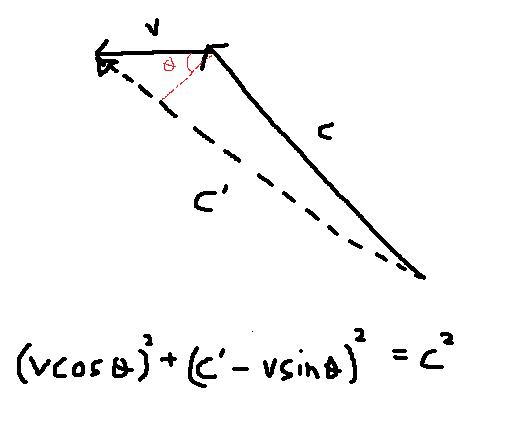Next pic shows the return trip: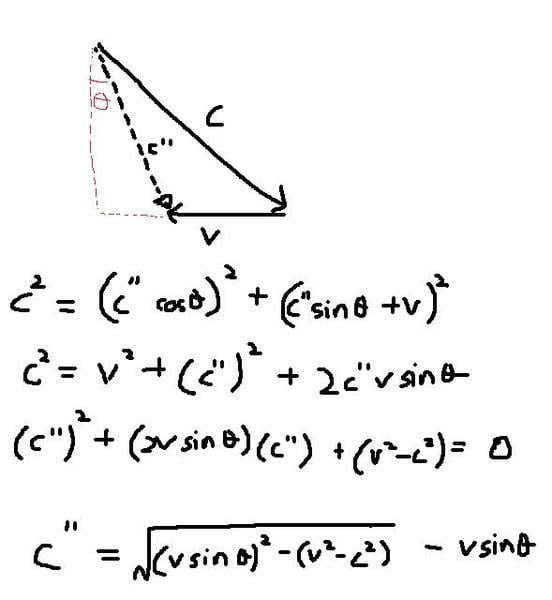Homework Helper
Gold Member

Everything looks good here. Just solve properly for c' and then simplify the expressions for t1 and t2.

unscientific

Everything looks good here. Just solve properly for c' and then simplify the expressions for t1 and t2.

I already did! And it got me:I'm thinking if i expanded t2 it will make things more complicated..

Homework Helper
Gold Member

Your expressions for c' and c" are not correct inside the brackets for t2, even though your expressions in your previous post are correct.

Last edited:
unscientific

You expressions for c' and c" are not correct inside the brackets for t2, even though your expressions in your previous post are correct.

I finally proved it! I simplified the expressions for t1 and t2 and found that the squareroots reduced to just 1... thanks for your help!

Homework Helper
Gold Member

Good Work!